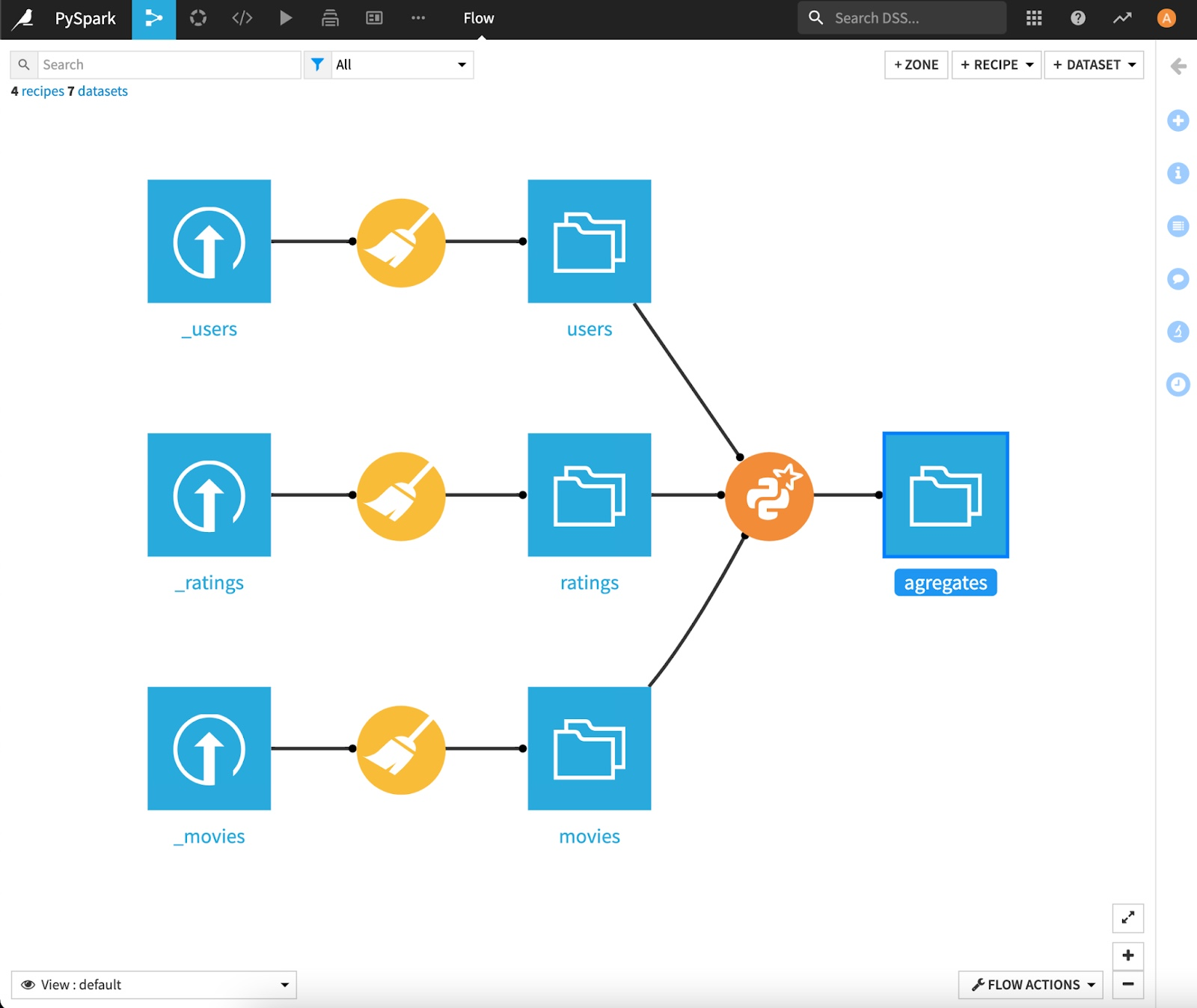# Using PySpark in DSS¶

Out of the numerous ways to interact with Spark, the DataFrames API, introduced back in Spark 1.3, offers a very convenient way to do data science on Spark using Python (thanks to the PySpark module), as it emulates several functions from the widely used Pandas package. Let’s see how to do that in Dataiku DSS.

## Prerequisites¶

You’ll need access to an instance of Dataiku with Spark enabled, and a working installation of Spark, version 1.4+.

We’ll use the MovieLens 1M dataset, made of 3 parts: ratings, movies and users. You can start with downloading and creating these datasets in Dataiku, and parse them using a Visual Data Preparation script to make them suitable for analysis: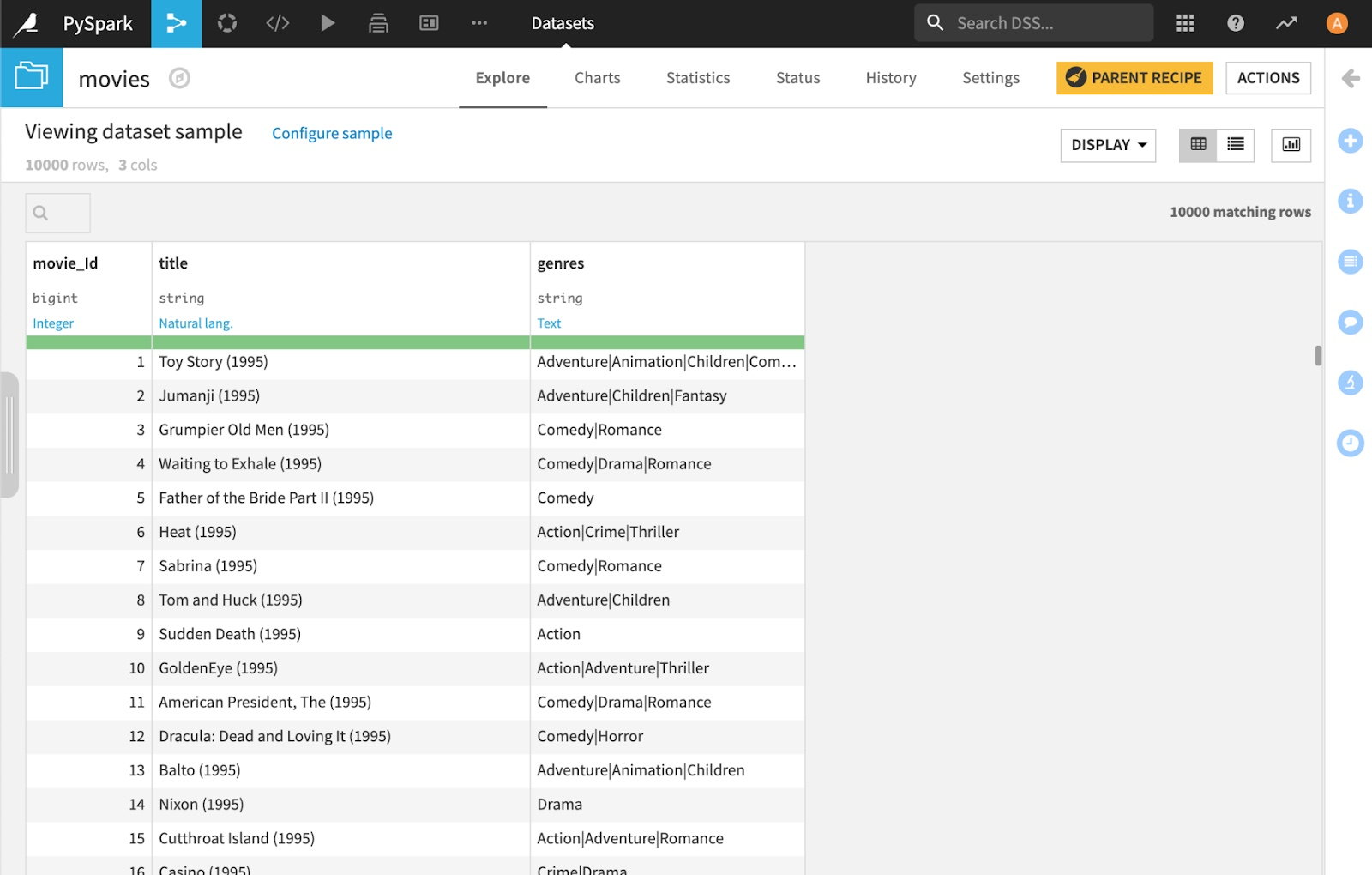You should end up with 3 datasets:

• movies

• users

• ratings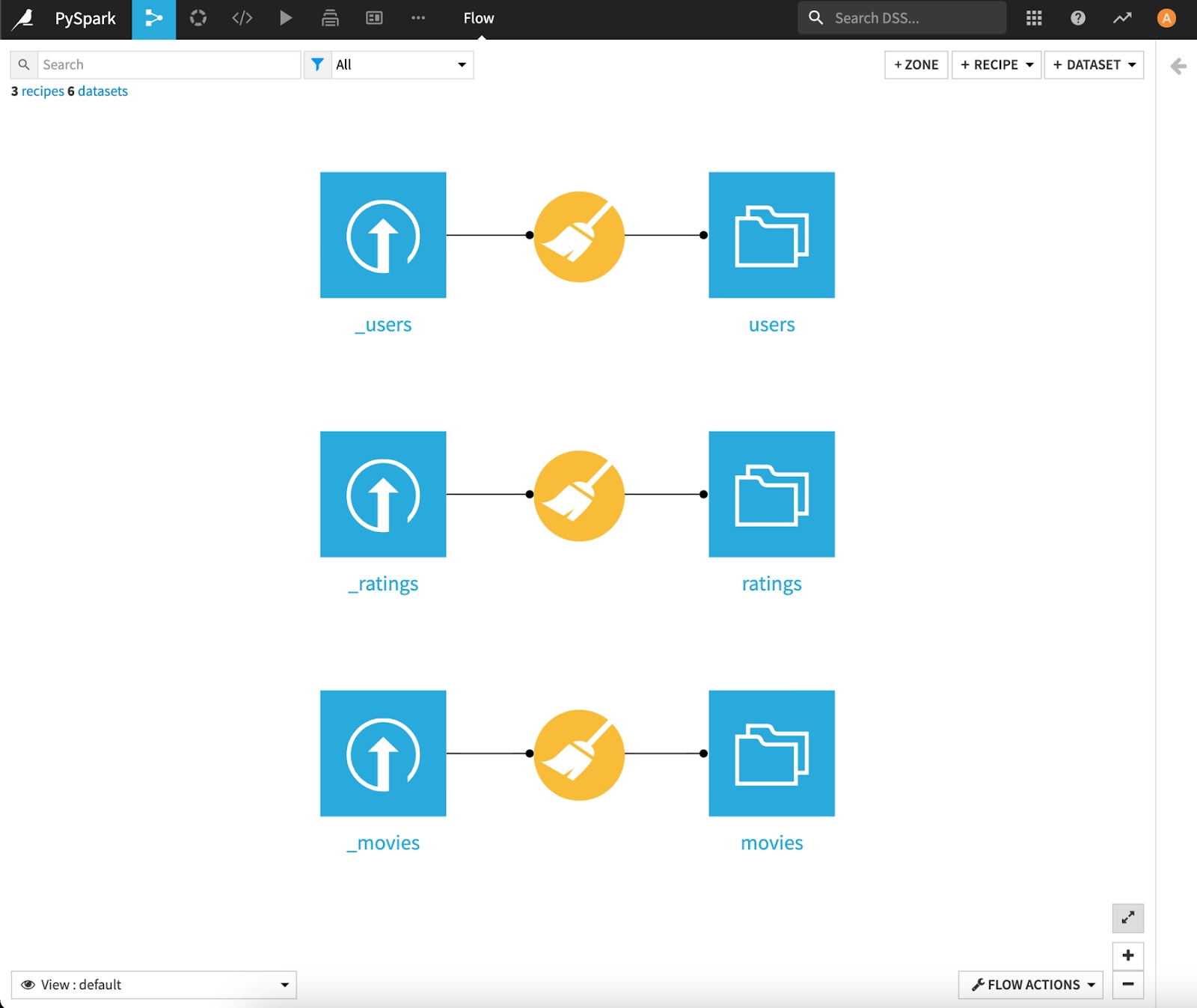## Creating DataFrames using PySpark and Dataiku APIs¶

As with regular Python, one can use Jupyter, directly embedded in Dataiku, to analyze interactively its datasets.

Go to the Notebook section from the Dataiku top navbar, click New Notebook, and choose Python. In the new modal window showing up, select Template: Starter code with PySpark: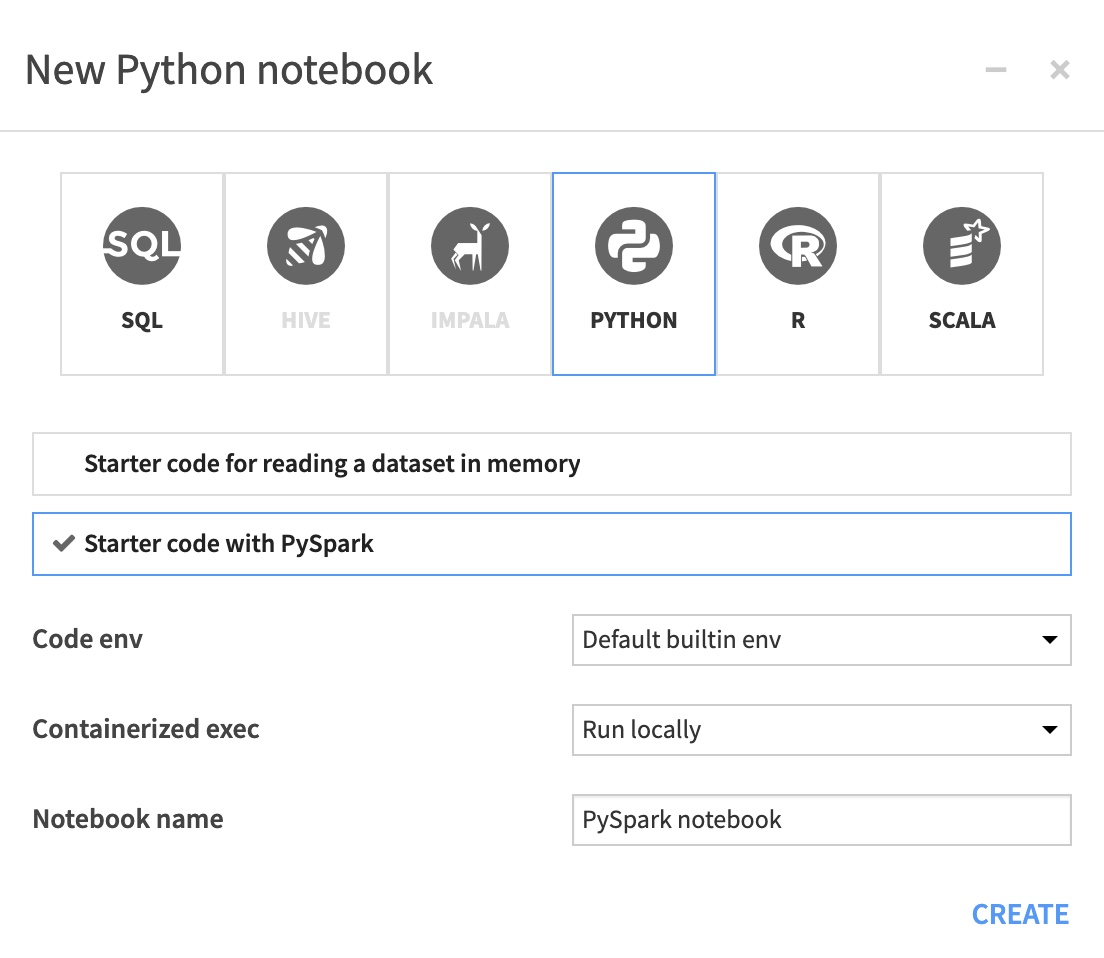You are taken to a new Jupyter notebook, conveniently filled with starter code: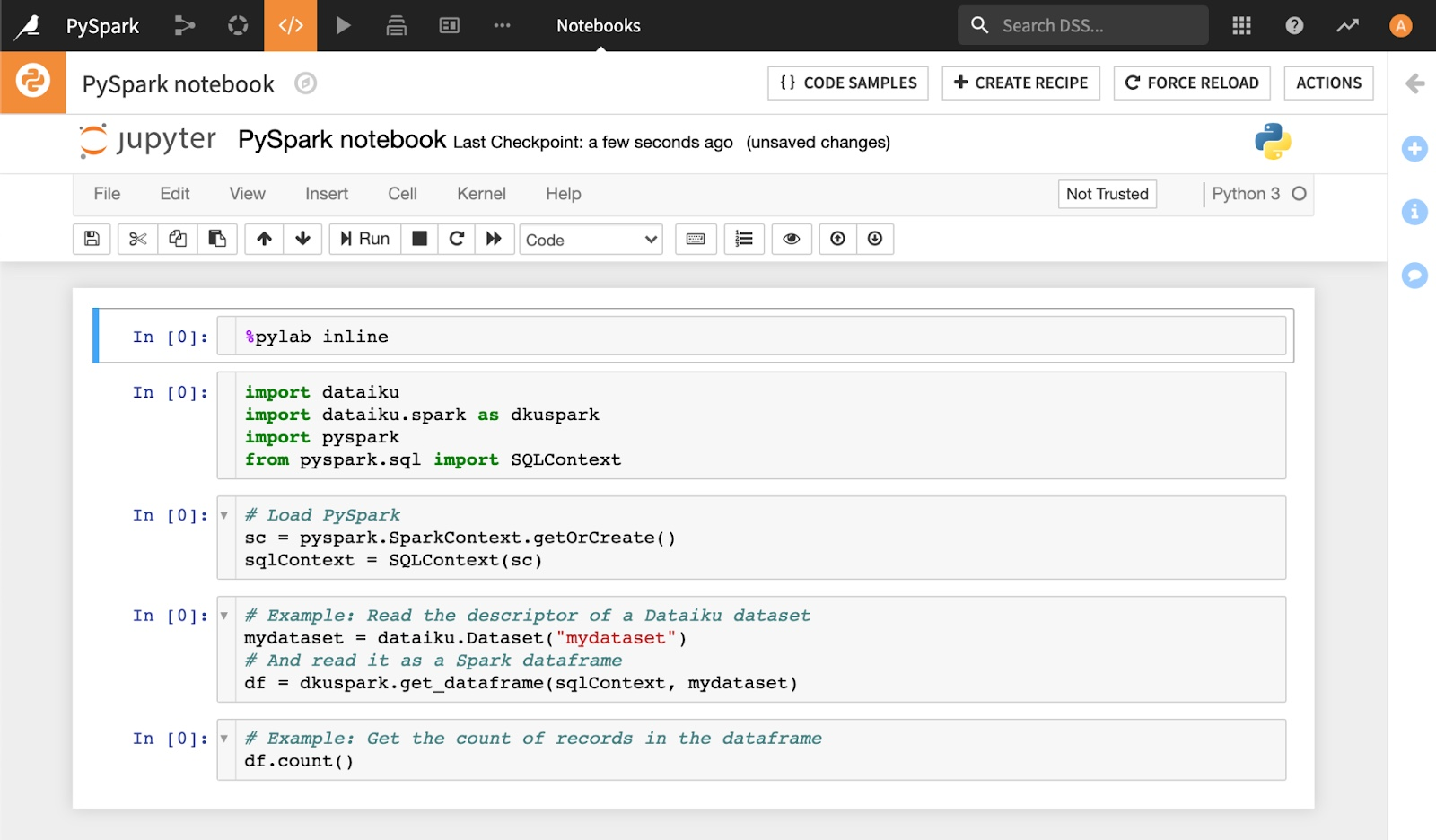Dataiku does the heavy lifting in terms of “plumbing”, so loading the Datasets into DataFrames is as easy as typing the following lines of code in your Jupyter notebook:

# Dataiku and Spark Python API's
import dataiku
import dataiku.spark as dkuspark
import pyspark
from pyspark.sql import SQLContext

sc = pyspark.SparkContext()
sqlContext = SQLContext(sc)

# Point to the DSS datasets
users_ds = dataiku.Dataset("users")
movies_ds = dataiku.Dataset("movies")
ratings_ds = dataiku.Dataset("ratings")

# And read them as a Spark dataframes
users = dkuspark.get_dataframe(sqlContext, users_ds)
movies = dkuspark.get_dataframe(sqlContext, movies_ds)
ratings = dkuspark.get_dataframe(sqlContext, ratings_ds)


The hardest part is done. You can now start using your DataFrames using the regular Spark API.

A Spark DataFrame has several interesting methods to uncover their content. For instance, let’s have a look at the number of records in each dataset:

print("DataFrame users has %i records" % users.count())
print("DataFrame movies has %i records" % movies.count())
print("DataFrame ratings has %i records" % ratings.count())


DataFrame users has 6040 records, DataFrame movies has 3883 records, and DataFrame ratings has 1000209 records.

You can also look at the actual content of your dataset using the .show() method:

users.show()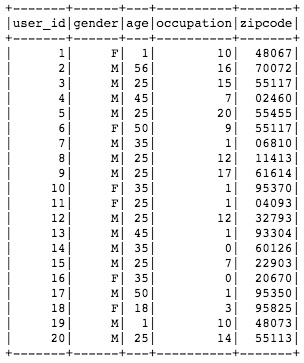You may want to only check the column names in your DataFrame using the columns attribute:

dfs = [users, movies, ratings]

for df in dfs:
print(df.columns)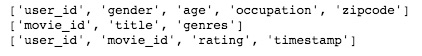The printSchema() method gives more details about the DataFrame’s schema and structure:

print(users.printSchema())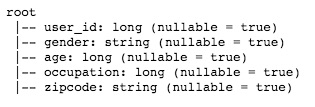Note

The DataFrame schema directly inherits from the Dataiku Dataset schema, which comes very handy when you need to manage centrally your datasets and the associated metadata!

Let’s have a look now at more advanced functions. Let’s start with merging the datasets together to offer a consolidated view.

a = ratings\
.join(users, ratings['user_id']==users['user_id'], 'inner')\
.drop(users['user_id'])

complete = a\
.join(movies, a['movie_id']==movies['movie_id'], 'inner')\
.drop(movies['movie_id'])

print(complete.count())
print(complete.show())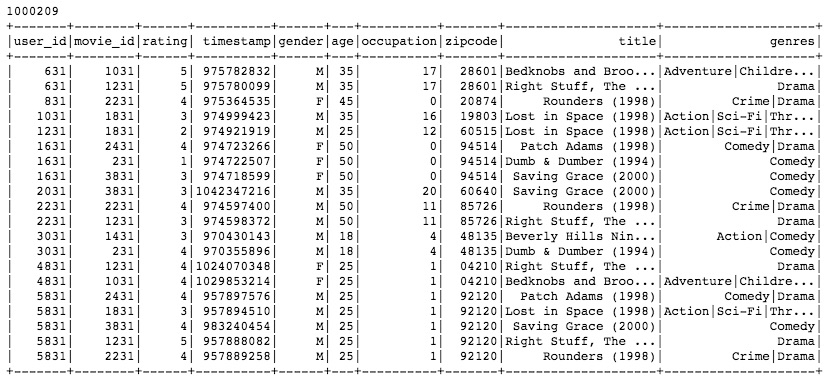Let’s assume you need to rescale the users ratings by removing their average rating value:

from pyspark.sql import functions as spfun

# Computing the average rating by user
avgs = complete.groupBy('user_id').agg(
spfun.avg('rating').alias('avg_rating')
)

# Join again with initial data
final = complete\
.join(avgs, complete['user_id']==avgs['user_id'])\
.drop(avgs['user_id'])

# Create a new column storing the rescaled rating
df = final.withColumn('rescaled_rating', final['rating'] - final['avg_rating'])


How do the rescaled ratings differ by occupation code? Don’t forget that you are in a regular Jupyter / Python session, meaning you can use non-Spark functionalities to analyze your data.

matplotlib.style.use('ggplot')

# Spark DataFrame
stats = df.groupBy('occupation').avg('rescaled_rating').toPandas()

# Pandas dataframe
stats.columns = ['occupation', 'rescaled_rating']
stats = stats.sort_values('rescaled_rating', ascending=True)

stats.plot(
kind='barh',
x='occupation',
y='rescaled_rating',
figsize=(12, 8)
)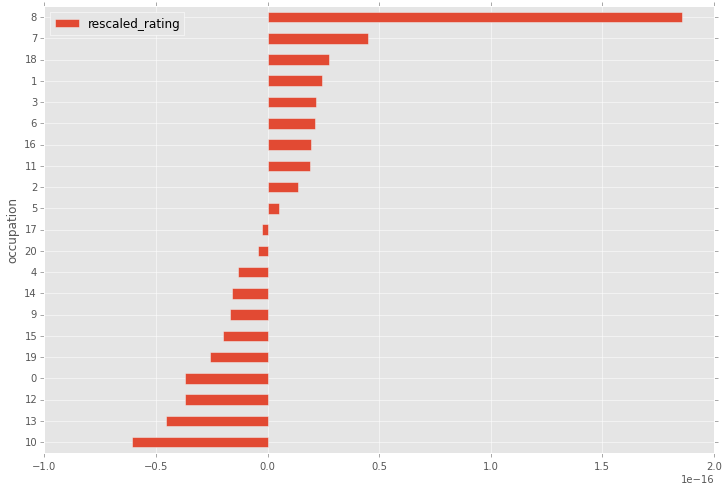## Creating PySpark recipes to automate your workflow¶

Finally, once your interactive session is over and you are happy with the results, you may want to automate your workflow.

Go back to the Flow screen, select all three datasets (by holding down the Shift key), then choose PySpark from the right pane: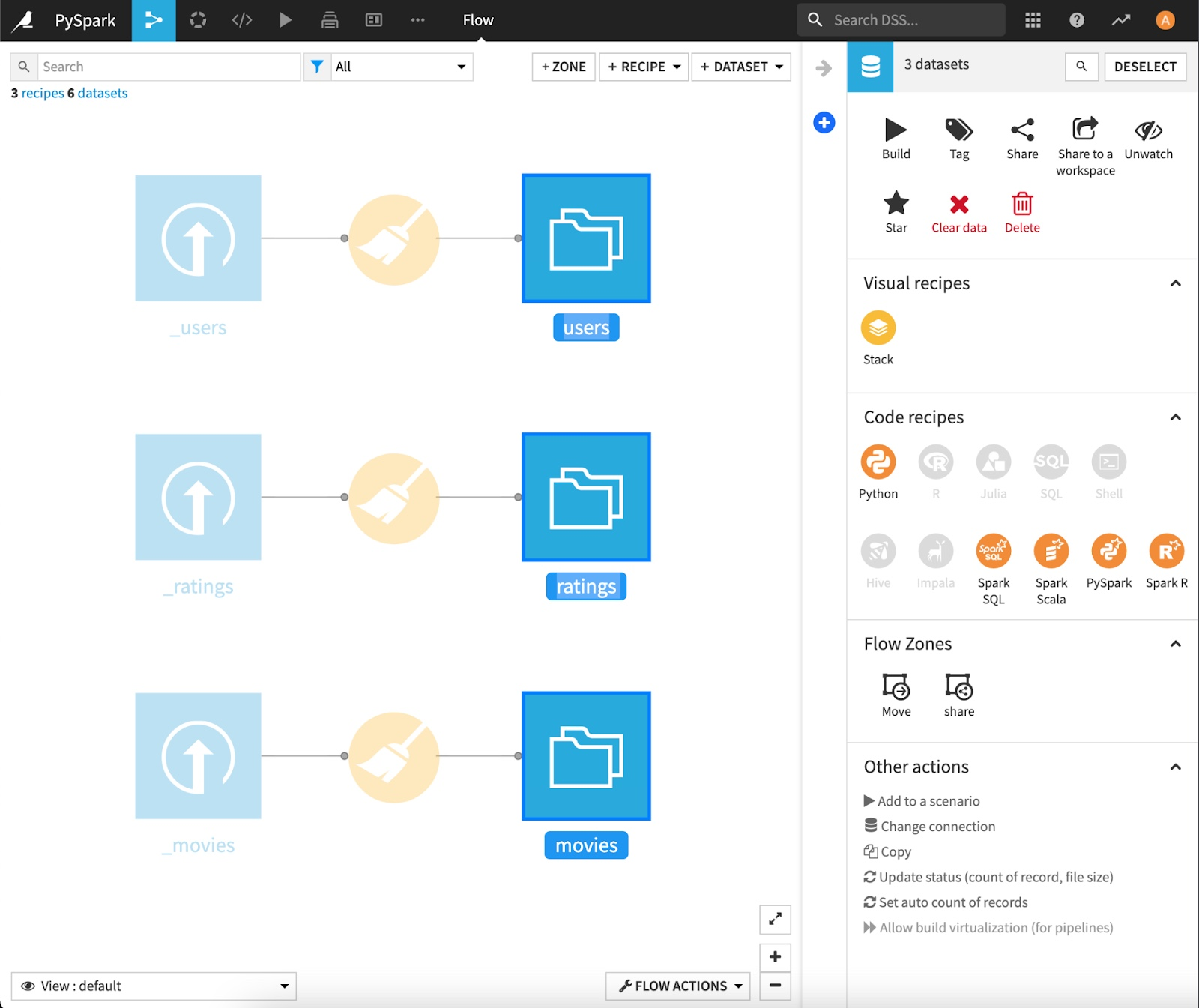Select the 3 MovieLens datasets as inputs, and create a new dataset called agregates on the machine filesystem: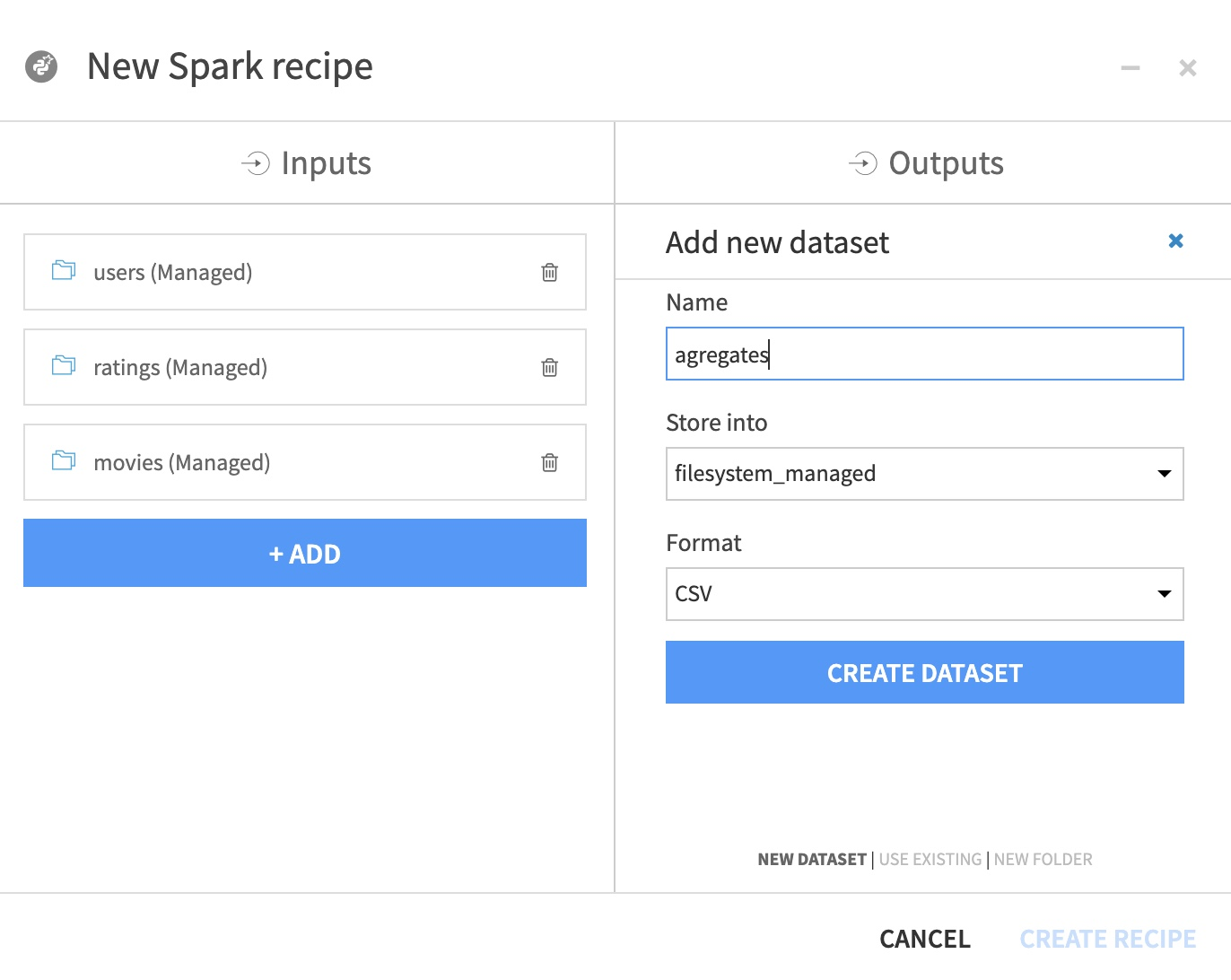In the recipe code editor, copy/paste the content of the downloaded Python file, and add the output dataset:

# -*- coding: utf-8 -*-
import dataiku
import dataiku.spark as dkuspark
import pyspark
from pyspark.sql import SQLContext
from pyspark.sql import functions as spfun

sc = pyspark.SparkContext()
sqlContext = SQLContext(sc)

# Point to the DSS datasets
users_ds = dataiku.Dataset("users")
movies_ds = dataiku.Dataset("movies")
ratings_ds = dataiku.Dataset("ratings")

# And read them as a Spark dataframes
users = dkuspark.get_dataframe(sqlContext, users_ds)
movies = dkuspark.get_dataframe(sqlContext, movies_ds)
ratings = dkuspark.get_dataframe(sqlContext, ratings_ds)

# Analysis
a = ratings\
.join(users, ratings['user_id']==users['user_id'], 'inner')\
.drop(users['user_id'])

complete = a\
.join(movies, a['movie_id']==movies['movie_id'], 'inner')\
.drop(movies['movie_id'])

avgs = complete.groupBy('user_id').agg(
spfun.avg('rating').alias('avg_rating')
)

final = complete\
.join(avgs, complete['user_id']==avgs['user_id'])\
.drop(avgs['user_id'])

df = final.withColumn('rescaled_rating', final['rating'] - final['avg_rating'])

stats = df.groupBy('occupation').avg('rescaled_rating')

# Output datasets
agregates = dataiku.Dataset("agregates")
dkuspark.write_with_schema(agregates, stats)


Hit the Run green button. The Spark jobs launches, and successfully completes (check your job’s logs to make sure everything went fine).